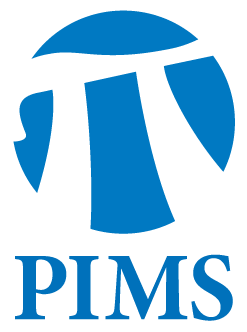## SCAIM Seminar: Nick Harvey

• Date: 11/22/2011
• Time: 12:30
Lecturer(s):
Nick Harvey
Location:

University of British Columbia

Topic:

Solving Laplacian Systems: Some Contributions from Theoretical Computer Science

Description:

Abstract:

This talk discusses algorithms to solve systems of linear equations where the matrix is the Laplacian matrix of a graph. These systems arise in many applications: in scientific computing, when using the finite difference method to approximately solve Poisson's equation; in machine learning, in some methods for semi-supervised learning on graphs; and in theoretical computer science, in fast algorithms for network flow problems.For two decades, theoretical computer scientists have been developing algorithms with provable running-time bounds for solving such systems of equations. The current state-of-the-art algorithm computes a solution with relative error epsilon in the energy norm in running time O(m log n (log log n)^2 log(1/epsilon)) for any Laplacian matrix of size n x n with m non-zero entries.These algorithms use several sophisticated tools, including low-stretch trees and concentration of random matrices. In this talk we give a survey of these results.------------- To (un)subscribe to the SCAIM mailing list, send email to majordomo@cs.ubc.ca with the line "(un)subscribe scaim".

Other Information:

Location: WMAX 110